﻿ Show Me The Physics Website - Intro to Friction Notes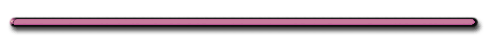HomeFriction

D) Friction - force between 2 surfaces that opposes motionDepends only on: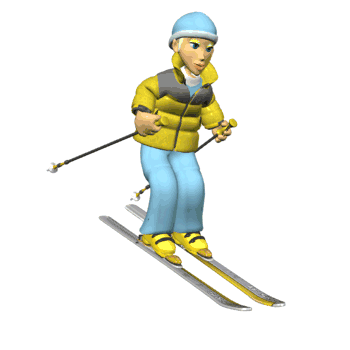1) texture of each surface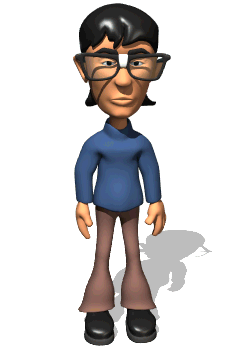What do plows have on their wheels to increase friction?

Chains

2) force holding objects togetherWhat do race cars have mounted on their rear hood to increase their friction?Spoilers increase the force between tire and roadWhy do some people put barbells in their car trunk on a snowy day?

Weights in a car truck
increase the tires force on the road

Two Kinds of Friction

(fs) 1) Static Friction - force of friction that must be overcome to move an object at rest.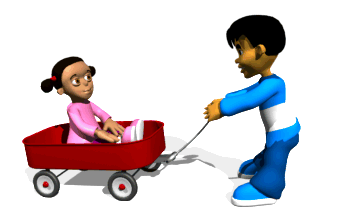(fk) 2) Kinetic Friction - force of friction that opposes a moving object.

Kinetic friction is weaker than static friction

fk < fs

Friction Equations

Static friction: fs = usFn

Kinetic friction: fk = ukFn

fs = force of static friction

fk = force of kinetic friction

Fn = normal force (supporting force)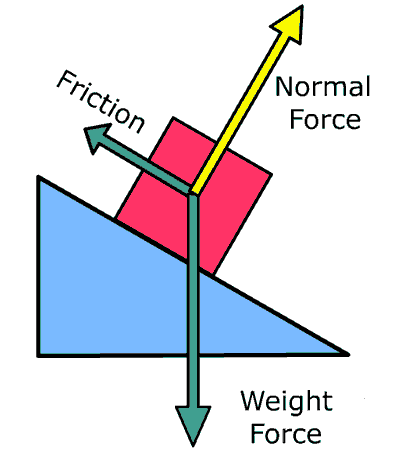us = coefficient of static friction - "the roughness number"

u
k = coefficient of kinetic friction - "the roughness number"

1. Relates to texture of surfaces in contact

2. Calculated in lab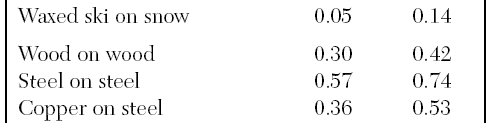ice and skate - low ukWhat do skiers do to their skis to lower the coefficient of friction between their skis and the snow?

Skiers put wax on the bottom
of their skis to lower the coefficient of friction

What is the direction of the friction at P?